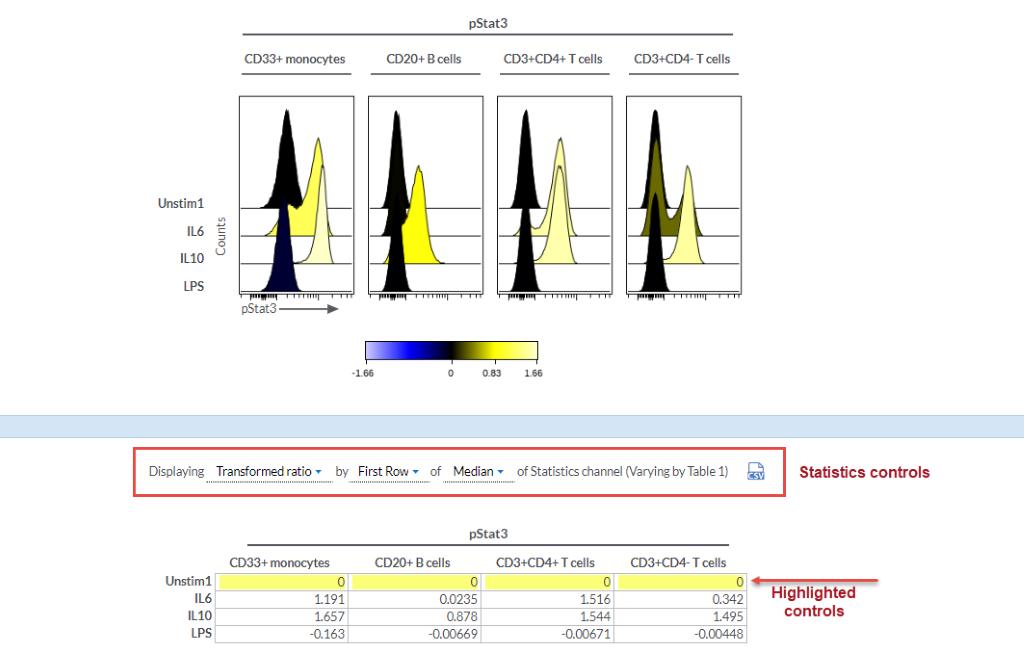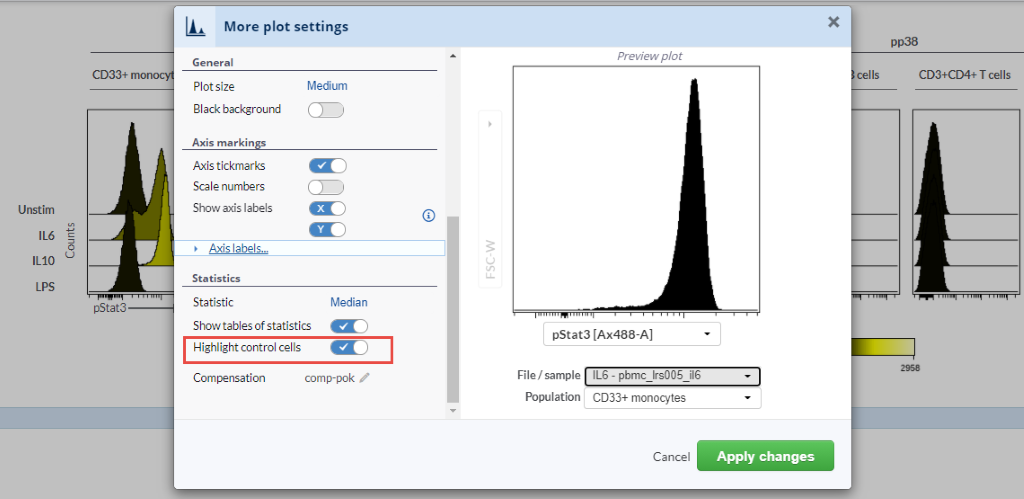Statistics and fold change equations in the Illustration Editior

Background

In the Cytobank platform, the Illustration Editor is used to dynamically create figures to explore data. The Illustration Editor provides both graphical and numerical summaries of the data in the form of a visual figure output and statistical output that corresponds to the data being visualized. The statistics for an illustration appear below the plots in the table of statistics and mimic the structure of the illustration itself.

The statistical output of the illustration can be customized in various ways. Click to jump to the section.

1. The statistic (e.g. median, mean, percentile)
2. The equation (e.g. raw or comparison between two samples)
3. The comparison control(e.g. baseline for comparison equations)

Anatomy(Illustration Editor statistics controls and example output)

Statistics available

• Median
• Percentile
• Mean
• Coefficient of Variation
• Percent in Gate
• Percent of Population
• Standard deviation
• Variance
• Minimum
• Maximum
• Event Count
• Channel Range
• Geometric Mean
• Percentile distance (5 to 95)
• Pearson correlation (X vs Y)

Equations to compare statistical data points

To compare samples with your statistic, you will need to select an equation for calculating the comparison. The available equations are described below. In each of these equations, x and control represent the statistic (indicated above) calculated on the data selected in the Illustration Editor or the comparison control, respectively.

1. Raw (no comparison between data points)

For each statistic you will see the raw, untransformed data values, without any comparison to other data points. Currently there is not an option to display transformed raw statistical values. Notes on a workaround to this are at the following link in the article on heatmaps.

Value = x

2. Log10 Ratio

Each data point in the table of statistics reports the log10 ratio of itself compared to its control.

Value = log10(x/control)

or written differently...

Value = log10(x) - log10(control)

3. Fold

Each data point in the table of statistics reports the raw fold ratio of itself compared to its control. Data values are not transformed for the calculation.

Value = x / control

4. Log2 Ratio

Each data point in the table of statistics reports the log2 ratio of itself compared to its control.

Value = log2(x / control)

or written differently...

Value = log2(x) - log2(control)

5. Arcsinh Ratio

Each data point in the table of statistics reports the arcsinh ratio of itself compared to its control.

Value = arcinsh(x / scale_argument) - arcsinh(control / scale_argument)

The scale argument ("cofactor") is taken according to the scale settings in the experiment. Even if the channel is not scaled by the arcsinh equation, the arcsinh ratio will still use arcsinh with the scale argument that is present in the scale editor at the time of the calculation.

6. Transformed Ratio

Each data point in the table of statistics reports the transformed ratio of itself compared to its control. The transformed ratio uses the scale settings of the channel being calculated.

Value = log10 ratio, arcsinh ratio, or fold, depending on scale settings. See sections above for an explanation of each equation.

Using the transformed ratio is recommended when you are calculating comparisons for any statistics that are affected by the transformations applied to the data, since the ratio that will be used is taken from the scale transformations applied to the entire experiment.

7. Difference

Each data point in the table of statistics reports the raw difference of itself and its control.

Value = x – control

8. Percentile distance (5 to 95)

scale_transformed (95th percentile) - scale_transformed (5th percentile)

Comparison control

When your goal is to compare samples with your statistic, a comparison control needs to be selected (as well as an equation, described below). Click on any of the plots, go to More plot settings, click the toggle to highlight the controls in the output statistics table and aid in interpretation (see example in image below).There are multiple options for control:

• First Row
• Column's Minimum
• Column's Maximum
• First Column
• Row's Minimum
• Row's Maximum
• Table's First Cell
• Table's Minimum
• Table's Maximum

Have more questions? Submit a request

Have more questions? Submit a request